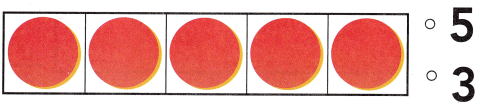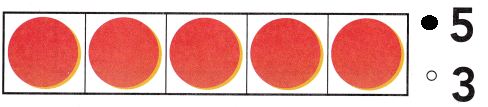# Texas Go Math Kindergarten Lesson 2.1 Answer Key Model and Count 5

Refer to our Texas Go Math Kindergarten Answer Key Pdf to score good marks in the exams. Test yourself by practicing the problems from Texas Go Math Kindergarten Lesson 2.1 Answer Key Model and Count 5.

## Texas Go Math Kindergarten Lesson 2.1 Answer Key Model and Count 5

Explore

DIRECTIONS: Place a counter on each orange as you count them. Move the counters to the five frame. Draw the counters.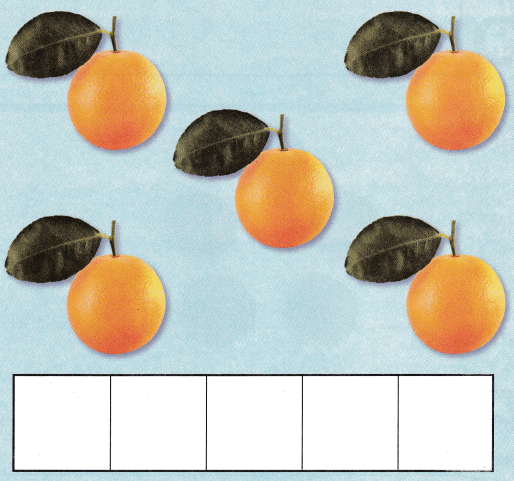Explanation:
Placed a counter on each orange as we count them.
Moved the counters to the five frame.

Share and Show

DIRECTIONS: 1-2. Place o counter on each object in the set as you count them. Move the counters to the five frame, Tell how many counters, Draw the counters.

Question 1.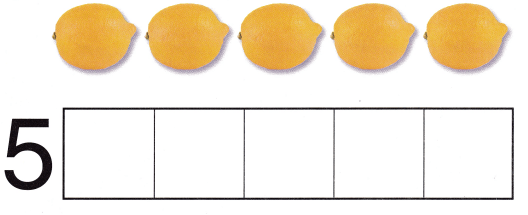Explanation:
Place a counter on each object in the set as we count them
and rewritten them on the frame

Question 2.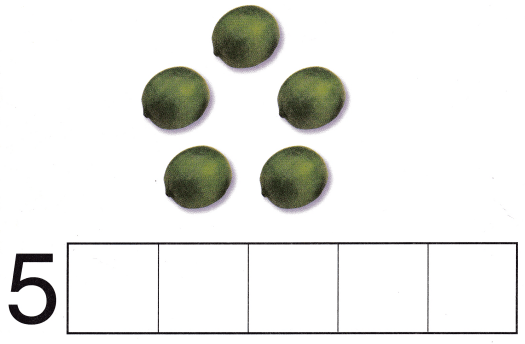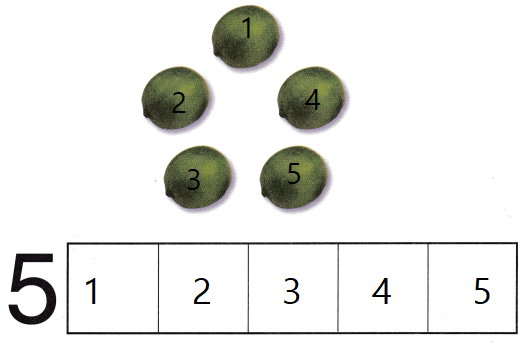Explanation:
Place a counter on each object in the set as we count them
and rewritten them on the frame

DIRECTIONS: 3. Place counters to show five. Draw the counters. 4. Place counters to show four. Draw the counters. Write the number. 5. Place counters to show five. Draw the counters. 6. Place counters to show three. Draw the counters. Write the number.

Question 3.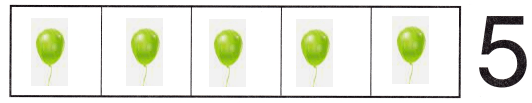Explanation:
Placed the counters to show  5

Question 4.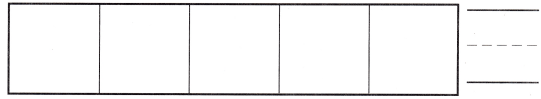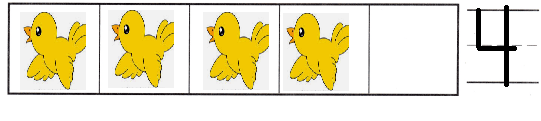Explanation:
Placed the counters to show 4

Question 5.Explanation:
Placed the counters to show 5

Question 6.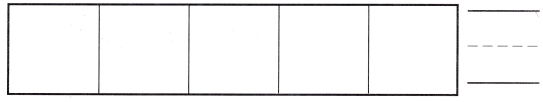Explanation:
Placed the counters to show 3

HOME ACTIVITY • Draw a five frame or use an egg carton with just five sections. Have your child show a set of five objects and place the objects in the five frame.Explanation:
Placed the counters to show 5

DIRECTIONS: 7. Count the objects in each set. Circle the sets of five objects. 8. Choose the correct answer. What number does the five frame show?

Problem Solving

Question 7.Explanation:
Placed the counters to show the 5 and circled them

Question 8.Explanation:
Counted the counters and bubbled the 5

### Texas Go Math Kindergarten Lesson 2.1 Homework and Practice Answer Key

DIRECTIONS: 1. Draw counters to show five. 2. Draw counters to show 3. Write the number, 3. Draw counters to show 4. Write the number.

Question 1.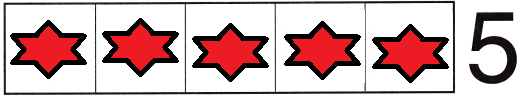Explanation:
Counted the counters and there  5
and drawn the counters

Question 2.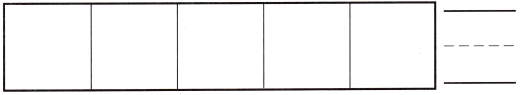Explanation:
Counted the counters and  there are 3
and drawn the counters

Question 3.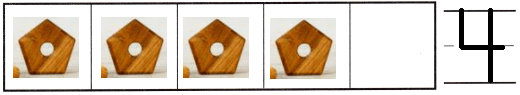Explanation:
Counted the counters and  there are 4
and drawn the counters

DIRECTIONS: 4-7. Choose the correct answer. What number does the five frame show?

Question 4.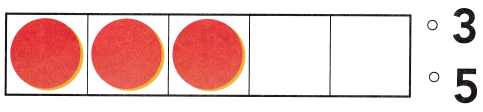Explanation:
Counted the counters and bubbled the 3

Question 5.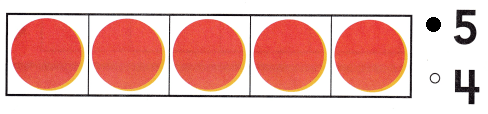Explanation:
Counted the counters and bubbled the 5

Question 6.